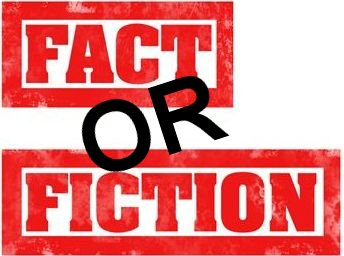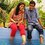# Is it $fact$$or$$fiction$ ?Is there any relation between both the given facts or this is coincidence : sum of $n$ natural numbers i.e. $\frac { n(n+1) }{ 2 }$ and ${n+1 \choose 2}$Note by Rishabh Jain
7 years, 1 month ago

This discussion board is a place to discuss our Daily Challenges and the math and science related to those challenges. Explanations are more than just a solution — they should explain the steps and thinking strategies that you used to obtain the solution. Comments should further the discussion of math and science.

When posting on Brilliant:

• Use the emojis to react to an explanation, whether you're congratulating a job well done , or just really confused .
• Ask specific questions about the challenge or the steps in somebody's explanation. Well-posed questions can add a lot to the discussion, but posting "I don't understand!" doesn't help anyone.
• Try to contribute something new to the discussion, whether it is an extension, generalization or other idea related to the challenge.

MarkdownAppears as
*italics* or _italics_ italics
**bold** or __bold__ bold
- bulleted- list
• bulleted
• list
1. numbered2. list
1. numbered
2. list
Note: you must add a full line of space before and after lists for them to show up correctly
paragraph 1paragraph 2

paragraph 1

paragraph 2

[example link](https://brilliant.org)example link
> This is a quote
This is a quote
    # I indented these lines
# 4 spaces, and now they show
# up as a code block.

print "hello world"
# I indented these lines
# 4 spaces, and now they show
# up as a code block.

print "hello world"
MathAppears as
Remember to wrap math in $$ ... $$ or $ ... $ to ensure proper formatting.
2 \times 3 $2 \times 3$
2^{34} $2^{34}$
a_{i-1} $a_{i-1}$
\frac{2}{3} $\frac{2}{3}$
\sqrt{2} $\sqrt{2}$
\sum_{i=1}^3 $\sum_{i=1}^3$
\sin \theta $\sin \theta$
\boxed{123} $\boxed{123}$

Sort by:

Yes There is relation between both If n(n+1)/2n When we have to find mean Then its equal to n+1/2

- 7 years, 1 month ago

- 7 years, 1 month ago

- 7 years, 1 month ago

Okay.

- 7 years, 1 month ago

- 7 years, 1 month ago

Yeah, I know. But he's looking for a reason aside from the coincidence, like a geometric or combinatorial proof. :P

- 7 years, 1 month ago

exactly !!

- 7 years, 1 month ago

No, I quite literally mean you answered the command given by Rishabh.

- 7 years, 1 month ago

If you factor $n+1$ choose $2$ you get $\dfrac{n(n+1)}{2!}$.

- 7 years, 1 month ago

oh! according to my knowledge $2!$$=$$2$ so what is the relation between the two

- 7 years, 1 month ago

Imagine the first one in a line of (n+1) persons saying "Hello" to all the n, second saying to remaining (n-1), third to rest of (n-2) and so on till the last but one says "Hello' to the last one. Total number of "Hello' is a combination term, 2 out of (n+1) as each and every pair said "Hello", which is also the sum of first n natural numbers.

- 7 years, 1 month ago